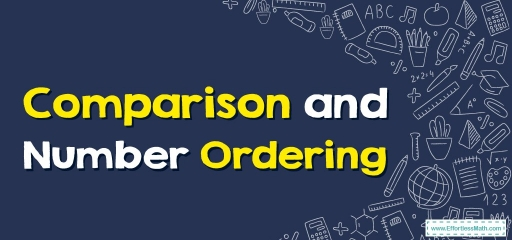# Comparison and Number Ordering

If you want to know how to compare and order numbers, the following article is for you.## What is comparison of numbers?

The method of finding out if a certain number is smaller than, bigger than, or equal to another number is known as number comparison.

## What is number ordering?

The method of placing certain numbers either in decreasing order (descending) or putting them in increasing order (ascending order) is known as number order.

## How does one do a comparison of  4-digit numbers?

The top crucial thing to understand whenever doing a comparison and ordering of numbers is what’s the value of the top most crucial digit in each one of those numbers.

The top crucial digit is going to allow you to know each number’s size. The top crucial digit for a number is the initial non-zero integer of that number. The bigger the digit, the larger the number is, except if it is negative.

Whenever doing a number comparison, many times we utilize symbols such as $$>, <$$ or $$=$$. Comparison in general, whenever $$a$$ and $$b$$ are $$2$$ numbers, the $$a >$$ signifies the number for $$a$$ is larger than the one for $$b$$;

$$a < b$$ indicates $$a$$ is smaller or less than $$b$$, and finally, $$a = b$$ indicates the number a is the same as the number for $$b$$.

You can use the following steps to discover what number is bigger or lesser.

Step One: Check the digits shown in the Thousands position, the number with a larger digit is bigger. If these $$2$$ numbers have the exact same amount of thousands, the next step is to do a comparison of the hundreds.

Step two: Check the digits in the Hundreds position, the number with a larger digit is bigger. If these numbers additionally have the identical amount of hundreds, the next thing to do is a comparison of the tens.

Step three: Check the digits in Tens position, the one with a larger digit is bigger. If they likewise have an equal amount of tens, you should do a comparison of the ones.

Step four: Check the digits in the One’s position, the number with a larger digit is bigger.

## How do we do a comparison of negative numbers?

A comparison of negative numbers is somewhat complex, however, the operation has been made simpler via these rules:

For the negative numbers, the more a number becomes the lower its value.

Step one: Moving to the right of the number line raises the negative number’s value.

Step two: Moving to the left alongside a number line, reduces the negative number’s value.

Step three: Zero as well as any type of positive number is constantly bigger than a number that’s negative.

## Comparison example – What number is larger: 3245 or 3284?

Firstly, we generate a place value chart for each of these numbers as seen in the following.

Step one: Check the number appearing in the Thousands position. Both digits are equal: $$3$$

Step two: Check the number appearing in the Hundreds position. Both are equal: $$2$$

Step three: Check the number appearing in the Tens position. Eight is more than four. So $$3284$$ is more than $$3245$$ or you could say it as $$3245$$ is less than $$3284$$.

### Comparison and Number Ordering – Example 1:

Solve. Use less than, equal to or greater than.

$$-43$$______ $$-12$$

Solution:

In comparison numbers, if both numbers are negative, the larger the number, the smaller the value. So, $$-43$$ is less than $$-12$$

### Comparison and Number Ordering – Example 2:

Solve. Use less than, equal to or greater than.

$$72$$______ $$54$$

Solution:

In comparison numbers, if both numbers are positive, the larger the number, the greater the value. So, $$72$$ is greater than $$54$$

## Exercises for Comparison and Number Ordering

Solve. Use less than, equal to or greater than.

1. $$32$$_____$$-32$$
2. $$84$$_____$$74$$
3. $$-90$$_____$$-15$$
4. $$44$$_____$$55$$
1. greater than
2. greater than
3. less than
4. less than

### What people say about "Comparison and Number Ordering - Effortless Math: We Help Students Learn to LOVE Mathematics"?

No one replied yet.

X
30% OFF

Limited time only!

Save Over 30%

SAVE $5 It was$16.99 now it is \$11.99